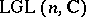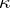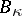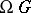# Birkhoff stratification

(diff) ← Older revision | Latest revision (diff) | Newer revision → (diff)
As an immediate consequence of Birkhoff factorization, [a1], the group of differentiable invertible matrix loopsmay be decomposed in a union of subsets, labelled by unordered-tuples of integers. Each of these consists of all loops withas the set of partial indices. This decomposition is called a Birkhoff stratification. It reflects important properties of holomorphic vector bundles over the Riemann sphere [a2], singular integral equations [a3], and Riemann–Hilbert problems [a4]. The structure of a Birkhoff stratification resembles those of Schubert decompositions of Grassmannians and Bruhat decompositions of complex Lie groups (cf. also Bruhat decomposition). The Birkhoff strataare complex submanifolds of finite codimension in. Codimension, homotopy type and cohomological fundamental class ofare expressible in terms of the label[a5]. The adjacencies among the Birkhoff strata describe deformations of holomorphic vector bundles [a5]. Birkhoff stratifications also exist for loop groups of compact Lie groups [a6]. For the group of based loopson a compact Lie group, the Birkhoff strata are contractible complex submanifolds labelled by the conjugacy classes of homomorphisms[a6]. Birkhoff stratification has a visual interpretation in the framework of Morse theory of the energy function on[a6]. Certain geometric aspects of Birkhoff stratification may be described in terms of non-commutative differential geometry and Fredholm structures [a7], [a8]. In particular, the Birkhoff strata become Fredholm submanifolds ofendowed with various Fredholm structures. Fredholm structures onarise from the natural Kähler structure on[a7] and in the context of generalized Riemann–Hilbert problems with coefficients in[a8]. Curvatures and characteristic classes of Birkhoff strata may be computed in the spirit of non-commutative differential geometry, in terms of regularized traces of appropriate Toeplitz operators [a7].